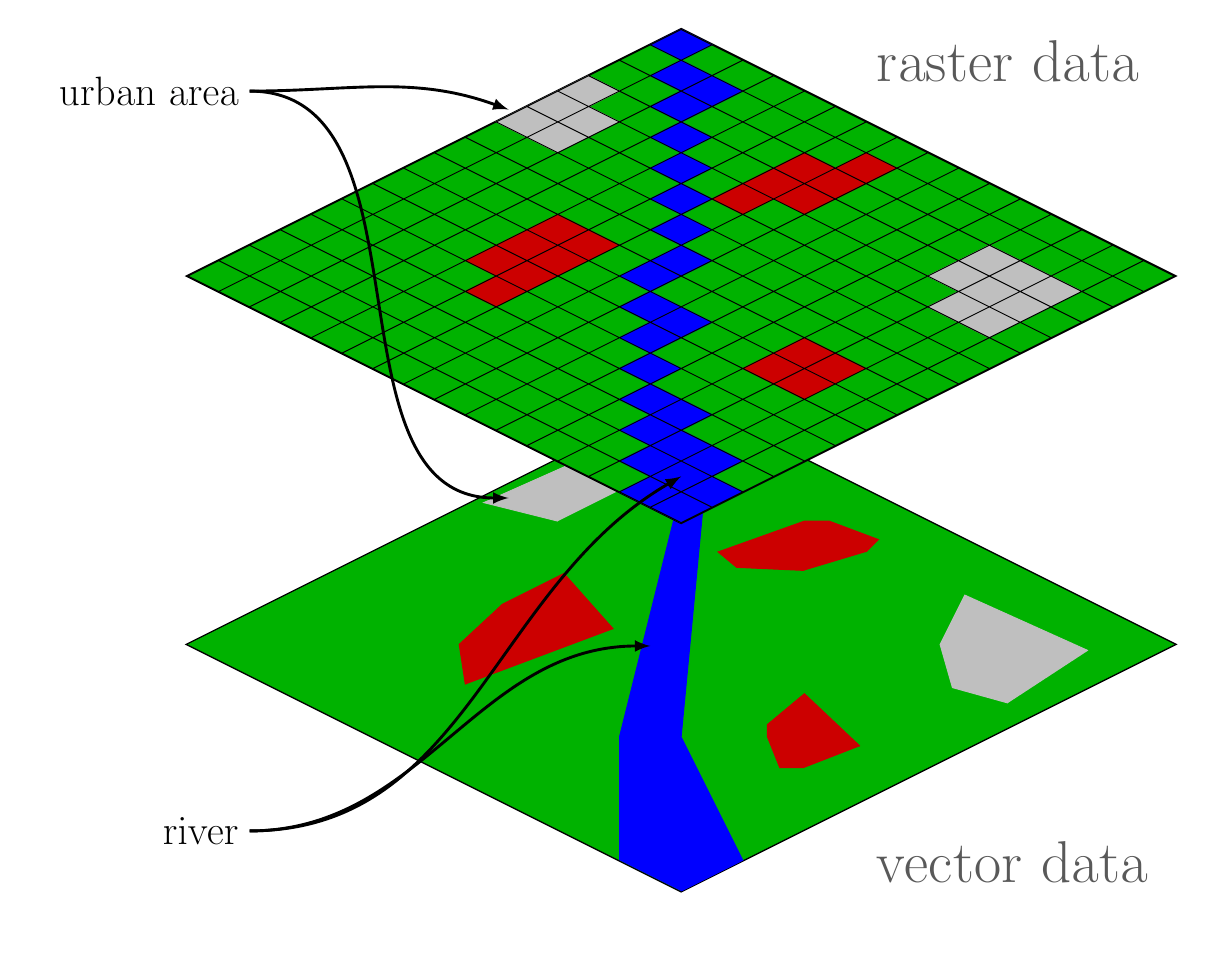# Geographic Data Structure: Vector Data and Raster Data

Geographic data are geographically referenced data wherein individuals can locate and identify features by a spherical coordinate system i.e. latitude and longitude. Based on the nature of the display, this data is classified into two models i.e. vector and raster.

## Vector Data

Vector data models use X and Y coordinates to define the geographic elements. It represents in the form of points, lines and polygons depending upon the feature. As such, vector data tend to define the centres and edges of certain features.

• Point Data – is represented by one pair of coordinates ( X and Y) and is considered a dimensionless object because it can neither measure its length nor breadth with its data set. It is most commonly used to represent non-adjacent features and discrete data points. For example, the location of a house, location of a well etc. (see figure 1)
• Line Data– It is used to represent the data that have linear features which contain at least two pairs of coordinates such as X1 Y1, X2 Y2. The start and endpoints are called nodes and the points on curves are called vertices. They are one dimensional. For example rivers, railway lines, roadways etc. (see figure 1)
• Polygon Data– Polygon data is represented as a closed line encompassing an area. It takes a minimum of three pairs of coordinates, i.e., X1Y1, X2Y2, X3Y3 to represent an area or polygon. It is two dimensional in nature. Polygon feature is mostly distinguished using thematic symbols or colours for visual representation. Examples- Forest area, Agricultural land, snow cover etc. (see figure 1)

## Raster Data

Raster data is synonyms with grid data as it consists of pixels with an array of cells. Pixels represent each and every geographic element. One pixel only contains one value. Hence, a number of raster layers are required for the representation of multiple features over a common plane. (see figure 1)

### Difference Between Vector Data and Raster Data

 Vector Data Raster Data 1. It is used to store data having discrete boundaries in Point, Line and Polygon 1. It consists of cells organised in rows and columns with each cell having one value. 2. It is discrete in nature. 2. It is continuous in nature. 3. It is complex data 3. It is simple data 4. Example- Administrative borders, roadways, rivers, location of a house, forest area, fallow land etc. 4. Example- Temperature, air pressure, elevation, flow and distance etc.Figure 1  Vector and Raster data representation

## Conclusion

Hence, there are some primary difference between Vector and Raster data. The vector data represents data using sequential points or vertices as points, lines and polygons. Whereas, the raster data represents a grid matrix. They both are the two types of spatial data in GIS.